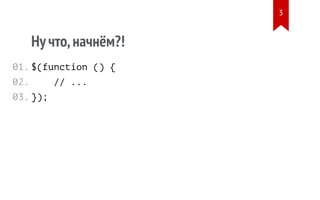Successfully reported this slideshow.

# "Observable и Computed на пример KnockoutJS", Ольга Кобец, MoscowJS 29

0

Share×
1 of 45
1 of 45

# "Observable и Computed на пример KnockoutJS", Ольга Кобец, MoscowJS 29

0

Share

Доклад Ольги "Observable и Computed на пример KnockoutJS" на MoscowJS 29

Доклад Ольги "Observable и Computed на пример KnockoutJS" на MoscowJS 29

## More Related Content

### Related Books

Free with a 14 day trial from Scribd

See all

### Related Audiobooks

Free with a 14 day trial from Scribd

See all

### "Observable и Computed на пример KnockoutJS", Ольга Кобец, MoscowJS 29

1. 1. Observable и Computed на пример KnockoutJS 1
2. 2. Hello, MoscowJS 29 2
3. 3. Ну что,начнём?! \$(function () { // ... }); 01. 02. 03. 3
5. 5. 1. Реактивное программирование 2. Observable и Сomputed 3. Заглянем под капот 4. Другие реализации 5
6. 6. Реактивное программирование 6
7. 7. 7 bit.ly/what-is-reactive
8. 8. “I have no ... idea” Erik Meijer 8
9. 9. // 3 // Hello, World var a = 1; var b = 2; var sum = a + b; console.log(sum); a = 'Hello, '; b = 'World'; console.log(sum); 01. 02. 03. 04. 05. 06. 07. 9
10. 10. 10
11. 11. 11
12. 12. Резюмируя • Деклативно описывать поведение • Автоматически обновлять зависимости 12
13. 13. Observable и Computed 13
14. 14. Observable • Поддерживает чтение и запись • Можно подписаться 14
15. 15. // Create // Write value // Read value var myObservable = ko.observable(); myObservable(42); myObservable(); 01. 02. 03. 04. 05. 06. 15
16. 16. // 42 Подписка на изменения var myObservable = ko.observable(); myObservable .subscribe(value => console.log(value)); myObservable(42); 01. 02. 03. 16
17. 17. Computed • Вычисляется из зависимостей • Поддерживает только чтение • Можно подписаться 17
18. 18. // Create // Read value // Subscribe to change var myComputed = ko.computed( () => myObservable() + 1 ); myComputed(); myComputed.subscribe(onChange); 01. 02. 03. 04. 05. 06. 07. 08. 18
19. 19. a() b() // 3 // Hello, 2 // Hello, World var a = ko.observable(1); var b = ko.observable(2); var sum = ko.computed(() => + ); console.log(sum()); sum.subscribe(value => console.log(value)); a('Hello, '); b('World'); 01. 02. 03. 04. 05. 06. 07. 19
20. 20. 20
21. 21. Задача • Выводить список операций • Обновлять его по таймеру 21
22. 22. 22
23. 23. key insert update Хранение данных view.operations = ko.collection({ : data => data.id, : data => new Operation(data), : (item, data) => item.data(data) }); 01. 02. 03. 04. 05. 23
24. 24. Операция function Operation(data) { var view = this; view.id = data.id; view.data = ko.observable(data); view.progress = ko.computed(() => { return view.data().progress; }; } 01. 02. 03. 04. 05. 06. 07. 08. 24
25. 25. Получение данных get('/operations') .then(data => view.operations(data)); 01. 02. 25
26. 26. Ещё • Добавить сортировку 26
27. 27. 27
28. 28. view.operation() view.sort() Хранение данных view.sort = ko.observable('id'); view.columns = { id: item => item.id, progress: item => item.progress() } view.sortedOperations = ko.computed(() => { return sort( , ); }); 01. 02. 03. 04. 05. 06. 07. 08. 28
29. 29. Ещё • Добавить фильтр по id 29
30. 30. 30
31. 31. view.sortedOperation() view.filter() Хранение данных view.filter = ko.observable(''); view.filteredOperations = ko.computed(() => { return filter( , ); }); 01. 02. 03. 04. 05. 06. 07. 31
32. 32. XHR operations sortedOp filteredOp sort filter DOM 32
33. 33. 33
34. 34. Заглянем под капот 34
35. 35. a() Пример var a = ko.observable(); var b = ko.computed(() => + 1); 01. 02. 35
36. 36. Подписка var a = ko.observable(); a.subscribe(value => console.log(value)); ----> a._subscribers.push({ callback: callback }); 01. 02. 36
37. 37. Оповещение a(42); ----> a._notifySubscribers(); ----> a._subscribers.callback(); // 42 37
38. 38. Вычислительный контекст • Стек из контекстов • Регистрация в контексте 38
39. 39. Чтение и регистрация a(); ----> ko._context.register(a); 39
40. 40. Создание контекста var b = ko.computed(() => a() + 1;); ----> ko._context.create(b); ----> b._evaluate(); ----> ko._context.register(a); ----> a.subscribe(() => b._evaluate();); ----> ko._context.remove(); 40
41. 41. Создание computed 1. Вызываем конструктор Computed 2. Computed создаёт контекст 3. Knockout вычисляет Computed 4. Computed подписывается на зависимости 5. Computed удаляет вычислительный контекст 41
42. 42. Другие реализации 42
43. 43. Reactive Extentions • Пример bit.ly/rx-example • Доклад bit.ly/rx-talk 43
44. 44. Elm • Пример bit.ly/elm-example • Доклад bit.ly/elm-talk 44
45. 45. Спасибо за внимание. Вопросы? • Оля Кобец, Яндекс • Twitter @homyasusina • Пример bit.ly/ko-example • Слайды bit.ly/homyasusina-talk 45